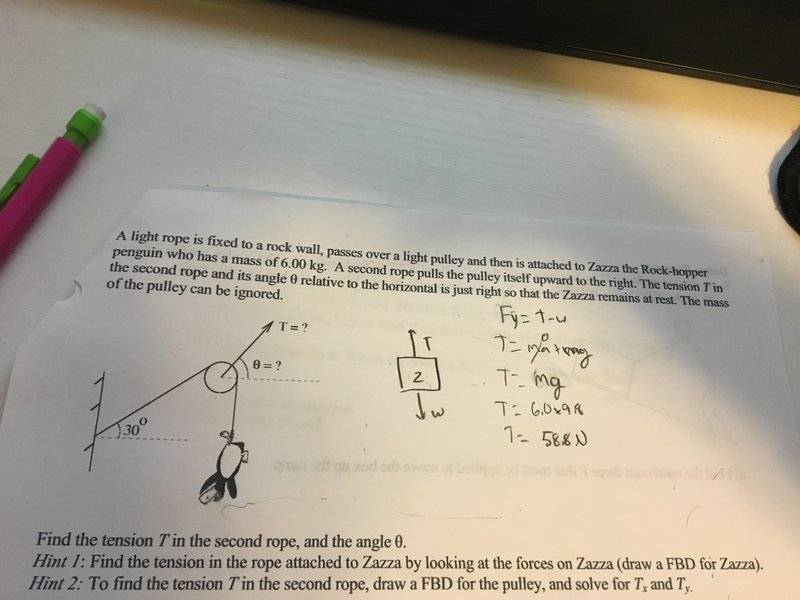# Need help with a pulley and tension problem

• Scott McKinnon
In summary, the problem is trying to find the tension in the horizontal component of the second rope's tension and finding the angle theta.f

## Homework Statement

https://imgur.com/KANlHh9The problem and my equation for finding the tension in rope one are shown in the image above. I am stuck on finding the tension for the horizontal component of the second rope's tension and finding the angle theta

F=ma
F=T-W

## The Attempt at a Solution

[/B]
To find the tension in rope 2 I have tried to find the vertical component first, here's a run-down of what I did.

Fy=T2y-Wy
ma=T2y-mg
0=T2y-mg (since the penguin is at rest a=0)
T2y=mg
T2y=6.00kg*9.8m/s^2
T2y=58.8N

And for the horizontal component, I think the equation would go something like this?

Fx=T2x?

For angle theta I need more than one component to solve for, so at this point I can't, but if I had the x and y compenents I could just use tan^-1 adj/opp.

#### Attachments

Last edited by a moderator:
It is very hard to read your mathematical symbols. There's a guide for writing nicer mathematics using LaTex: https://www.physicsforums.com/help/latexhelp/

But you haven't considered all the forces in the x-direction and the y-direction. There are two objects of interest: the penguin and the pulley.

On the penguin, we have two forces: A rope pulling up, and gravity pulling down. So there is no x-component. The forces have to add to zero.

On the pulley, we have three forces: One rope pulling straight down. One rope pulling at an angle of ##30^o##. And one rope pulling at angle ##\theta##. So you need to figure out the forces in the x-direction and the forces in the y-direction separately.

•Marco Noguez
It will be very usefull to draw the Force Body Diagram of the pulley and answer the following question: What do you know about each tension force (magnitude and angle)?

The problem I have with reading your attempt is that you have not defined your variables. Which are you thinking of as rope 2?
If you mean the one at an angle then, as @Marco Noguez hints, your mistake is thinking of that tension as acting directly on the penguin. That rope is not attached to the penguin. Treat the pulley and penguin separately.